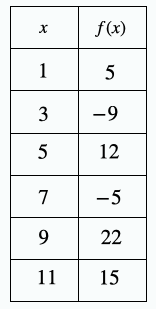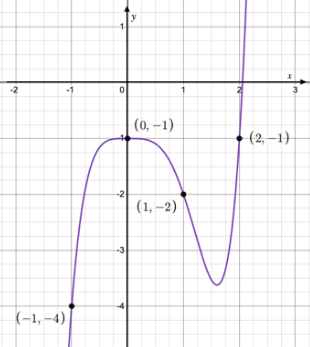# 8th Grade - Evaluating Functions

## Introduction

• Evaluating a function $f\left(x\right)$ means finding the value of the function that corresponds to a given input value of $x$.

## Evaluating functions

• The process of evaluating a function is described in the following two steps mentioned below:
• Replace the input variable with the provided value
• Simplify the expression to get the final result
• Sometimes a function can also be expressed in tabular form or graphical form. We find the output value in such cases by looking at the table or graph.

## Solved Examples

Question 1: Given $f\left(x\right)=2{x}^{2}-5x+1$, find the value of $f\left(3\right)$.

Solution: $f\left(x\right)=2{x}^{2}-5x+1$$=2×{\left(3\right)}^{2}-5×\left(3\right)+1$$=2×9-15+1$$=18-15+1$$=4$

Question 2: Based on the table below, find the value of $f\left(7\right)$.Solution: From the given table, we get $f\left(7\right)=-5$.

Question 3: Find $f\left(-1\right)$ from the graph shown below.Solution: From the given graph, we get $f\left(-1\right)=-4$.

## Cheat Sheet

• To evaluate a function at a given input, we substitute the variable in the function with the given input value and then simplify it to get the final result.

## Blunder Areas

• It is recommended to put the substituted values inside parentheses in order to avoid committing mistakes.
• Note that $f\left(x+a\right)\ne f\left(x\right)+f\left(a\right)$.